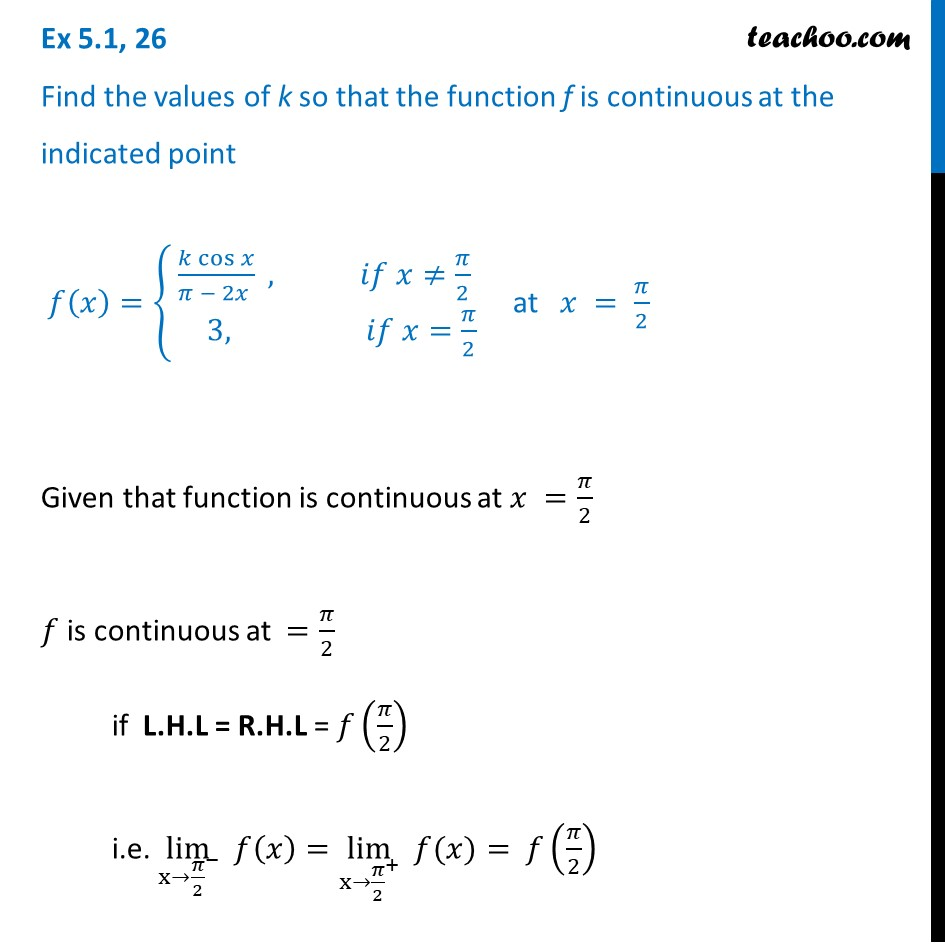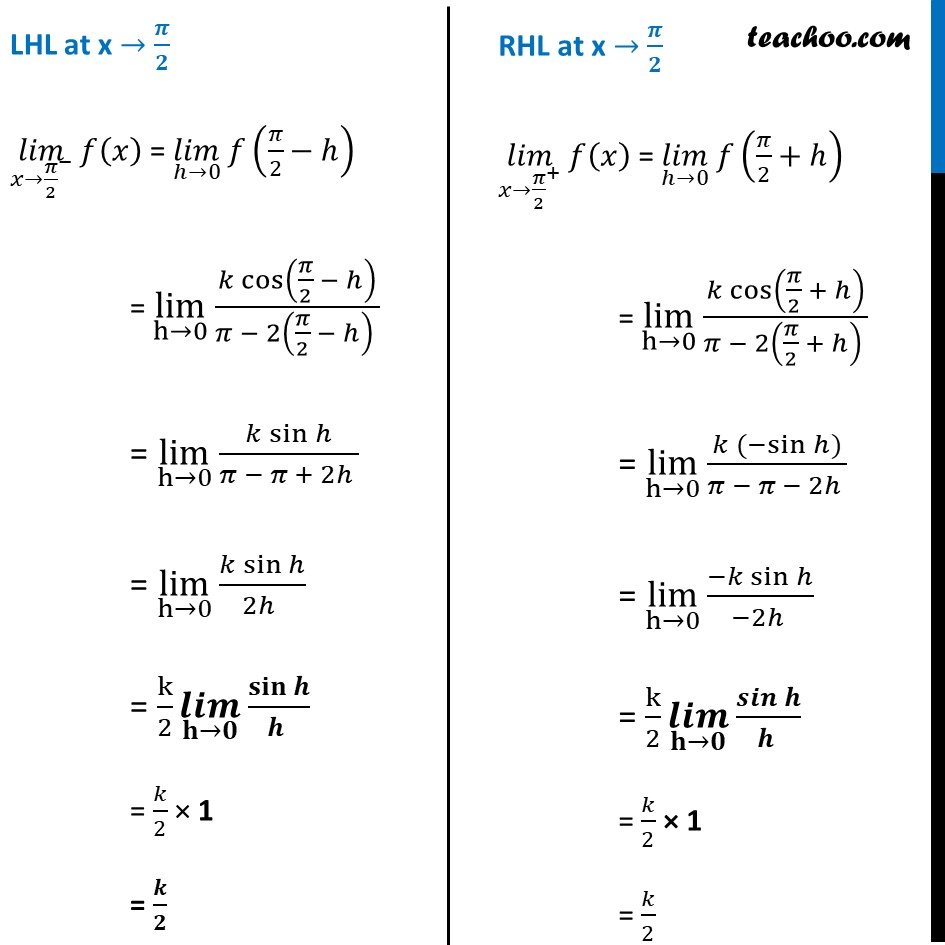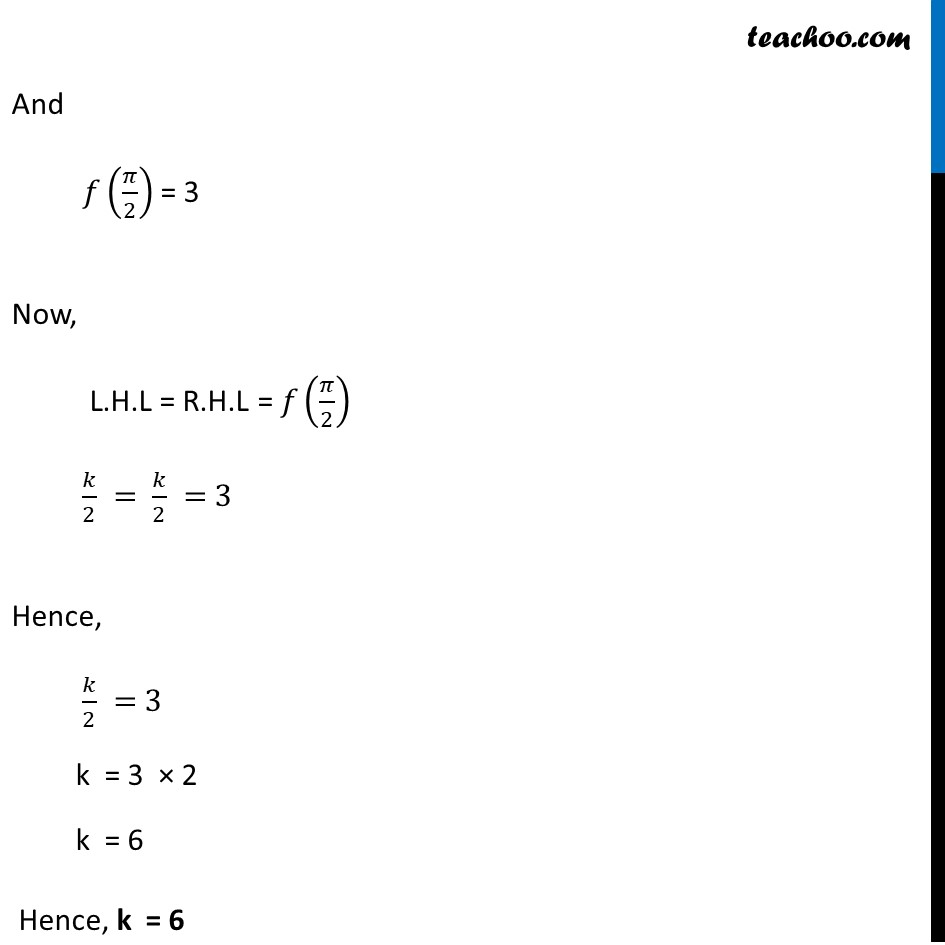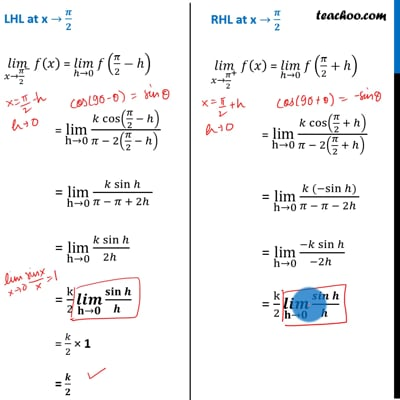Ex 5.1

Chapter 5 Class 12 Continuity and Differentiability
Serial order wiseThis video is only available for Teachoo black users

Solve all your doubts with Teachoo Black (new monthly pack available now!)

### Transcript

Ex 5.1, 26 Find the values of k so that the function f is continuous at the indicated point 𝑓(𝑥)={█((𝑘 cos⁡𝑥)/(𝜋 − 2𝑥 ) , 𝑖𝑓 𝑥≠𝜋/2@& 3, 𝑖𝑓 𝑥=𝜋/2)┤ at 𝑥 = 𝜋/2 Given that function is continuous at 𝑥 =𝜋/2 𝑓 is continuous at =𝜋/2 if L.H.L = R.H.L = 𝑓(𝜋/2) i.e. lim┬(x→〖𝜋/2〗^− ) 𝑓(𝑥)=lim┬(x→〖𝜋/2〗^+ ) " " 𝑓(𝑥)= 𝑓(𝜋/2) LHL at x → 𝝅/𝟐 (𝑙𝑖𝑚)┬(𝑥→〖𝜋/2〗^− ) 𝑓(𝑥) = (𝑙𝑖𝑚)┬(ℎ→0) 𝑓(𝜋/2−ℎ) = lim┬(h→0) (𝑘 cos⁡(𝜋/2 − ℎ))/(𝜋 − 2(𝜋/2 − ℎ) ) = lim┬(h→0) (𝑘 sin⁡ℎ)/(𝜋 − 𝜋 + 2ℎ ) = lim┬(h→0) (𝑘 sin⁡ℎ)/(2ℎ ) = k/2 (𝒍𝒊𝒎)┬(𝐡→𝟎) 𝐬𝐢𝐧⁡𝒉/(𝒉 ) = 𝑘/2 × 1 = 𝒌/𝟐 RHL at x → 𝝅/𝟐 (𝑙𝑖𝑚)┬(𝑥→〖𝜋/2〗^+ ) 𝑓(𝑥) = (𝑙𝑖𝑚)┬(ℎ→0) 𝑓(𝜋/2+ℎ) = lim┬(h→0) (𝑘 cos⁡(𝜋/2 + ℎ))/(𝜋 − 2(𝜋/2 + ℎ) ) = lim┬(h→0) (𝑘 〖(−sin〗⁡ℎ))/(𝜋 − 𝜋 − 2ℎ ) = lim┬(h→0) (−𝑘 sin⁡ℎ)/(−2ℎ ) = k/2 (𝒍𝒊𝒎)┬(𝐡→𝟎) 𝒔𝒊𝒏⁡𝒉/(𝒉 ) = 𝑘/2 × 1 = 𝑘/2 And 𝑓(𝜋/2) = 3 Now, L.H.L = R.H.L = 𝑓(𝜋/2) 𝑘/2 = 𝑘/2 = 3 Hence, 𝑘/2 = 3 k = 3 × 2 k = 6 Hence, k = 6